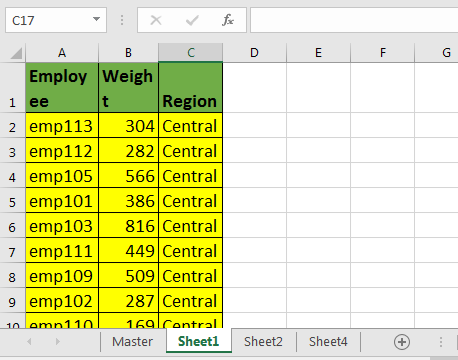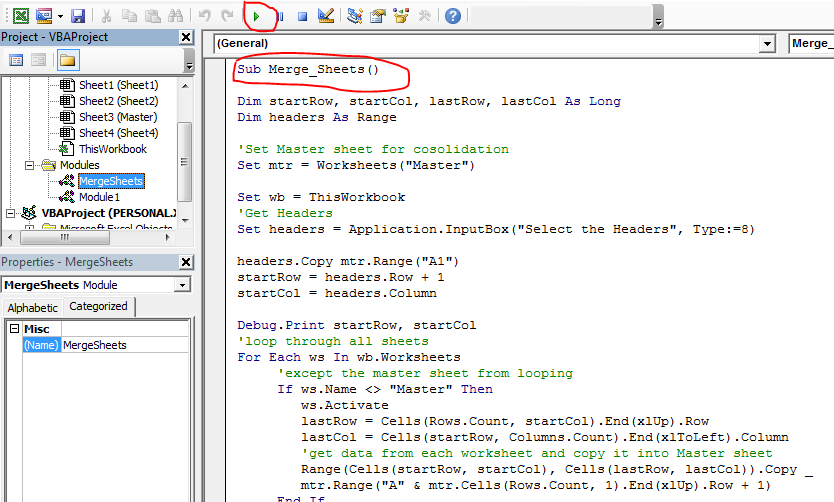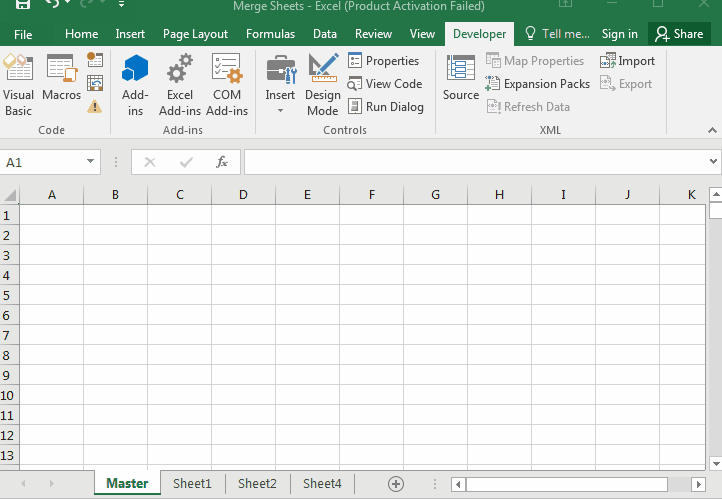# Consolidate/Merge multiple worksheets into one master sheet using VBA

Sometimes we want to merge multiple sheets into one sheet so that we can easily analyse the data and turn it into some useful information. This articles will tell you how to merge multiple worksheets into one worksheet using VBA.
Example:Here I have fetched some data from server that returns data into different worksheets. I have added one more sheet and named it as “Master”. Other sheet names doesn’t matter.
Now run this macro.

```Sub Merge_Sheets()

Dim startRow, startCol, lastRow, lastCol As Long

'Set Master sheet for consolidation
Set mtr = Worksheets("Master")

Set wb = ThisWorkbook

Debug.Print startRow, startCol
'loop through all sheets
For Each ws In wb.Worksheets
'except the master sheet from looping
If ws.Name <> "Master" Then
ws.Activate
lastRow = Cells(Rows.Count, startCol).End(xlUp).Row
lastCol = Cells(startRow, Columns.Count).End(xlToLeft).Column
'get data from each worksheet and copy it into Master sheet
Range(Cells(startRow, startCol), Cells(lastRow, lastCol)).Copy _
mtr.Range("A" & mtr.Cells(Rows.Count, 1).End(xlUp).Row + 1)
End If
Next ws

Worksheets("Master").Activate

End Sub
```

How to merge sheets using this VBA Macro?

1. Insert a new sheet and name it “Master” in the workbook. Rename it later if you want.
2. Insert a module in VBA editor and copy above VBA code.
3.4. Run the macro.

And it is done. All the sheets are merged in master.How it works?
I assume that you know the basics of object and variable creation in VBA. in the first part we have created object and variables that we will need in our operations.

Well most of the things I have explained using comments in vba code. Let’s look at the main part of this vba code.

```For Each ws In wb.Worksheets
'except the master sheet from looping
If ws.Name <> "Master" Then
ws.Activate
lastRow = Cells(Rows.Count, startCol).End(xlUp).Row
lastCol = Cells(startRow, Columns.Count).End(xlToLeft).Column
'get data from each worksheet and copy it into Master sheet
Range(Cells(startRow, startCol), Cells(lastRow, lastCol)).Copy _
mtr.Range("A" & mtr.Cells(Rows.Count, 1).End(xlUp).Row + 1)
End If
Next ws```

In earlier articles we learned how to loop through sheets and how to get last row and column using vba.

Here we are looping through each sheet in main workbook using for loop.
For Each ws In wb.Worksheets

Then we exclude “master” sheet from looping, since we will be consolidating our data in that sheet.

Then we get last row and last column number.

Now next line is very important. We have done multiple operations into one line.
Range(Cells(startRow, startCol), Cells(lastRow, lastCol)).Copy _
mtr.Range("A" & mtr.Cells(Rows.Count, 1).End(xlUp).Row + 1)

First we form a range using startRow, startCol and lastRow and lastCol.

```Range(Cells(startRow, startCol), Cells(lastRow, lastCol))
We have copied it using copy method of range.
Range(Cells(startRow, startCol), Cells(lastRow, lastCol)).Copy
We pasted it directly into first blank cell after last non blank cell in column A of master sheet (mtr.Cells(Rows.Count, 1).End(xlUp).Row + 1).

Range(Cells(startRow, startCol), Cells(lastRow, lastCol)).Copy _
mtr.Range("A" & mtr.Cells(Rows.Count, 1).End(xlUp).Row + 1)```

This loops runs for all the sheets and copies each sheets data into master sheet.

Finally, in the end of the macro we activate the mastersheet to see the output.

So yeah guys, this is how you can merge every sheet in a workbook. Let me know if you have any query regarding this VBA code or any excel topic in the comments section below.

Related Articles:

How to loop through sheets

how to get last row and column using vba

Delete sheets without confirmation prompts using VBA in Microsoft Excel

Add And Save New Workbook Using VBA In Microsoft Excel 2016

Display A Message On The Excel VBA Status Bar

Turn Off Warning Messages Using VBA In Microsoft Excel 2016

Popular Articles:

The VLOOKUP Function in Excel

COUNTIF in Excel 2016

How to Use SUMIF Function in Excel

Terms and Conditions of use

The applications/code on this site are distributed as is and without warranties or liability. In no event shall the owner of the copyrights, or the authors of the applications/code be liable for any loss of profit, any problems or any damage resulting from the use or evaluation of the applications/code.